×#### Thank you for registering.

One of our academic counsellors will contact you within 1 working day.

Click to Chat

1800-1023-196

+91-120-4616500

CART 0

• 0

MY CART (5)

Use Coupon: CART20 and get 20% off on all online Study Material

ITEM
DETAILS
MRP
DISCOUNT
FINAL PRICE
Total Price: Rs.

There are no items in this cart.
Continue Shopping```Continuity in Interval

Table of Content

Introduction to Continuity in Interval

Continuity of some of the common functions

Continuity of Composite Functions

Introduction to Continuity in IntervalA real function 'f' is said to be continuous in an open interval (a, b) if it is continuous at every point in the interval (a, b). Function 'f' is said to be continuous for closed interval [a, b] if it is continuous ∀ x ∈ (a, b) and

limn→a+ f(x) = f(a) and  limn→b-  f(x) = f(b)

Here the limits of end points of interval are one sided limit while solving problems one does not evaluate continuity condition at all points in the interval but uses his elementary knowledge of the function to find points of discontinuity and if none exists then function is continuous in the entire interval.

Let us define a function h(x) given by

h(x) = f(x) for a < x < b

= g(x) for b < x < c

Where f(x) and g(x) are continuous in their respective intervals. Then continuity if h(x) is checked only at x = b, as it is the only point where h(x) changes from f(x) to g(x) and hence is a likely point of discontinuity.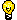Important

1. The necessary and sufficient condition for continuity of f at x = a ('a' being finite) is that both limx→a- f(x) and limn→a+ f(x) should exist and be equal to f(a)

2. If a function is continuous on a closed interval [a, b], a and b are necessarily finite, it is bounded on it. It may not be always true in case of open interval (a, b).

eg     f(x)= 1/x ∀ 0 < x ≤ 1

The functions is continuous but not bounded in the interval (0. 1)

f(x) is said to be continuous in an open interval (a, b) if it is continuous at every point in this interval. f(x) is said to be continuous in the closed interval [a, b] if

f(x) is continuous in (a, b)

limx→a+ f(x)=f(a)

limx→a- f(x)=f(a)

Illustration: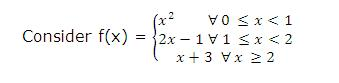Discuss the continuity of f(x)

Solution:

If we look at x2, 2x-1 or x+3, these functions are continuous in their respective intervals (Because every polynomial function is a continuous function). Hence we will check the continuity of f(x) at x=1 and x=2, because these are the points where the function is changing values. Consider x=1

limx→1+  f(x) = limh→0 (1-h)2 = 1

limx→1+  f(x) = limh→0  2 (1+h) - 1 = 1

f(1) = 2 - 1 - 1 = 1

Both limits equal to f(1), hence f(x) is continues at x=1

Consider x=2

limx→2-  f(x) = limh→0  2(2-h)-1 = 3

limx→2+  f(x) = limh→0 (2+h) + 3 - 5

f(2) = 2 + 3 = 5

Since LHL is not equal to f(2) hence f(x) is discontinuous at x = 2

Continuity of some of the common functions

Functions f(x)

Interval in which f(x) is continuous

Constant C

(-∞,∞)

bn, n is an integer > 0

(-∞,∞)

|x-a|

(-∞,∞)

x-n, n is a positive integer.

(-∞,∞)-{0}

a0xn + a1xn-1 +........ + an-1x + an

(-∞,∞)

p(x)/q(x), p(x) and q(x) are polynomials in x

R - {x:q(x)=0}

sin x

R

cos x

R

R-{(2n-1π)/2:n=0,±1,........}

tan x

R-{nπ:n=0,±1,........}

cot x

R-{(2n-1)π/2:n=0,±1,± 2,........}

sec x

R-{(2n-1)π/2:n=0,±1,± 2,........}

ex

R

Ln x

(0, ∞)

Illustration:

Let f(x)=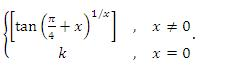For what value of k is f(x) continuous at x-0 ?

Solution: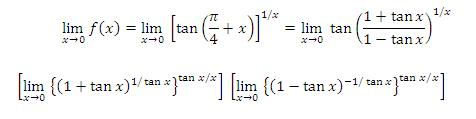= e × e = e2

Since f(x) is continuous at x = 0, limx→a  f(x) = f(0)

⇒ a2 = k

Hence f(x) is continuous at x = 0 when k = e2

Illustration:

Discuss the continuity of

f(x)=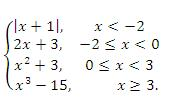Solution:

We rewrite f(x) as f(x) =As we can see, f(x) is defined as a polynomial function in each of the intervals (-∞, -2). (-2, 0), (0, 3), and (3, ∞). Therefore it is continuous in each of these four intervals.

At the point x = -2

limx→-2-  f(x)= limx→-2(-x-1)=1, and limx→-2+ f(x) = limx→-2+(2x+3)=-1

Therefore, limx→-2 f(x) does not exist.

Thus f(x) is discontinuous at x=-2. At a point x = 0

limx→0- f(x) = limx→0+ f(x) = f(0)=3,

Therefore f(x) is continuous at x=0. At a point x = 3

limx→3- f(x) = limx→3+ f(x) = f(3)=12

Therefore, f(a) is continuous at x=3.

So, we conclude that f(x) is continuous at all points in R except at x = -2.

Illustration:

Let f(x) be a continuous function and g(x) be a discontinuous function. Prove that f(x) + g(x) is a discontinuous function.

Solution:

Suppose that h(x) = f(x)+g(x) is continuous. Then, in view of the fact that f(x) is continuous, g(x)=h(x)-f(x), a difference of continuous functions, is continuous.

But this is a contraction since g(x) is given as a discontinuous function.

Hence h(x) = f(x) + g(x) is discontinuous.

Continuity of Composite Functions

If the function u = f(x) is continuous at the point x=a, and the function y=g(u) is continuous at the point u = f(a), then the composite function y=(gof)(x)=g(f(x)) is continuous at the point x=a.

Illustration:

Find the points of discontinuity of y = 1/(u2+u-2) where u= 1/(x-1)

Solution:

The function u = f(x)= 1/(x-1) is discontinuous at the point x=1.

The function y = g(u)= 1/(u2+u-2) = 1/((u+2)(u-1)) is discontinuous at

u = -2 and u =1.

⇒1/(x-1)=-2                 ⇒ x=1/2

When u = 1

⇒ 1/(x-1)=1                    ⇒x=2

Hence the composite function y = g(f(x)) is discontinuous at three  points  x = 1/2, x = 1 and x =2.
To read more, Buy study materials of Limtis and Continuity comprising study notes, revision notes, video lectures, previous year solved questions etc. Also browse for more study materials on Mathematics here.
```### Course Features

• 731 Video Lectures
• Revision Notes
• Previous Year Papers
• Mind Map
• Study Planner
• NCERT Solutions
• Discussion Forum
• Test paper with Video Solution